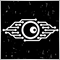460

Hi,
What does this indicator count for values?
It reminds me of RSI but it is a little different.

Thank you for help

``` double tempPrice;
double f88, f90;
double f0, v4, v8, vC, v10, v14, v18, v20;
double f8, f10, f18, f20, f28, f30, f38, f48, v1C;
double f50, f58, f60, f68, f70, f78, f80, f40;

//int counted_bars = IndicatorCounted(),limit,shift;

//if (counted_bars<0) return(-1);
//if (counted_bars>0) counted_bars--;
double limit=Bars-(Len)-1;

//if(counted_bars>Len) limit=Bars-counted_bars-1;

for (int shift=limit;shift>=0;shift--) {
tempPrice = GetPrice(PriceMode, shift);  // Get the price based on mode entered
if (f90 == 0.0) {

f90 = 1.0;
f0 = 0.0;
if (Len-1 >= 5) f88 = Len-1.0; else f88 = 5.0;
//      f8 = 100.0*(Close[shift]);
//
//      Modified for median price
//      Modified for any price based on PriceMode input
//      default input is typical price
//
//        f8 = 100.0*((High[shift]+Low[shift]+Close[shift])/3);
f8 = 100.0 * tempPrice;
f18 = 3.0 / (Len + 2.0);
f20 = 1.0 - f18;

} else{
if (f88 <= f90) f90 = f88 + 1; else f90 = f90 + 1;
f10 = f8;
//      f8 = 100*Close[shift];
//         f8 = 100.0*((High[shift]+Low[shift]+Close[shift])/3);
f8 = 100 * tempPrice;
v8 = f8 - f10;
f28 = f20 * f28 + f18 * v8;
f30 = f18 * f28 + f20 * f30;
vC = f28 * 1.5 - f30 * 0.5;
f38 = f20 * f38 + f18 * vC;
f40 = f18 * f38 + f20 * f40;
v10 = f38 * 1.5 - f40 * 0.5;
f48 = f20 * f48 + f18 * v10;
f50 = f18 * f48 + f20 * f50;
v14 = f48 * 1.5 - f50 * 0.5;
f58 = f20 * f58 + f18 * MathAbs(v8);
f60 = f18 * f58 + f20 * f60;
v18 = f58 * 1.5 - f60 * 0.5;
f68 = f20 * f68 + f18 * v18;

f70 = f18 * f68 + f20 * f70;
v1C = f68 * 1.5 - f70 * 0.5;
f78 = f20 * f78 + f18 * v1C;
f80 = f18 * f78 + f20 * f80;
v20 = f78 * 1.5 - f80 * 0.5;

if ((f88 >= f90) && (f8 != f10)) f0 = 1.0;
if ((f88 == f90) && (f0 == 0.0)) f90 = 0.0;
}

if ((f88 < f90) && (v20 > 0.0000000001)) {

v4 = (v14 / v20 + 1.0) * 50.0;
if (v4 > 100.0) v4 = 100.0;
if (v4 < 0.0) v4 = 0.0;

} else {
v4 = 50.0;
}```84991

Stanislav Milka:
Hi,